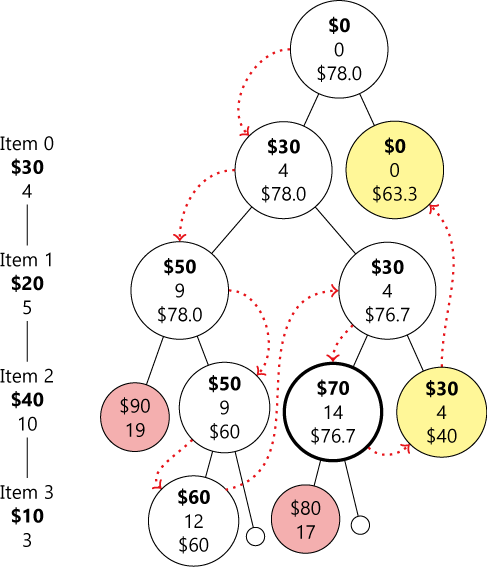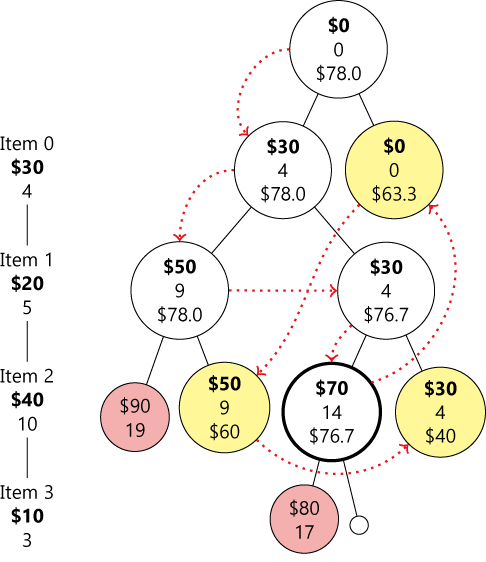# 0-1 背包问题详解 (4)from heapq import *

def knapsack(vw, limit):

def bound(v, w, j):
...

maxValue = 0
PQ = [[-bound(0, 0, 0), 0, 0, 0]]  # -bound to keep maxheap
while PQ:
b, v, w, j = heappop(PQ)
if b <= -maxValue:  # promising
if w + vw[j] <= limit:
maxValue = max(maxValue, v + vw[j])
heappush(PQ, [-bound(v + vw[j], w + vw[j], j + 1),
v + vw[j], w + vw[j], j + 1])
if j < len(vw) - 1:
heappush(PQ, [-bound(v, w, j + 1), v, w, j + 1])
return maxValue